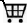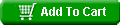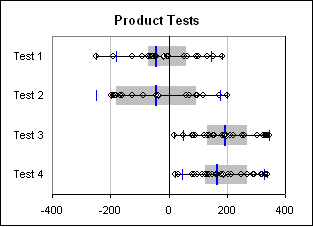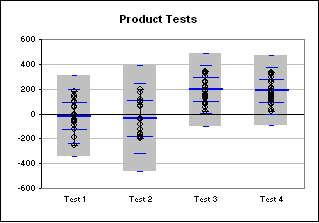Questions? E-mail us or call us 302-584-1771, 8AM to 10PM U.S. East Coast Time 7 days a week
 Home Products Add-in Collections Software Support Resources CompanyView Cart US \$29.95Box and Whisker Plot Creator An add-in product for Microsoft Excel Download a trial version now! Menu driven for ease of use Create quartile, percentile, or statistical box and whisker plots hide or show data points show 1-3 sigma bars on your plots Plot from multiple different worksheets or workbooks on the same box and whisker plot Screen shots:The Box and Whisker Plot Creator add-in for Microsoft Excel makes it easy to create box and whisker plots.  It is compatible with Microsoft Excel 2010-2021 and Office 365.

The following illustrates such charts created by the Box and Whisker Plot Creator.  The charts may be horizontal or vertical:

 Quartile box and whisker plot Statistical box and whisker plotThe first box and whisker plot above is a quartile plot  The gray area is where one expects 50% of the points to be.  The points outside of the gray to the left represents the first quartile, or 25% of the points. The points outside and to the right represents the remaining 25%.  The center blue bar is the median.  The average is plotted as a white diamond.  The smaller blue bars are the 1.5 sigma points.

The second box and whisker plot is a statistical plot.  The gray area in this case is the 3 sigma range. One can set the range to be 1, 2, or 3 sigma. The center blue bar in the second box and whisker plot is the average (as it is a statistical plot).  The three smaller sets of blue bars represent the 1, 2, and 3 sigma points.

On both plots one can:

• hide or show all the data points
• hide or show the sigma bars
• Show only outlier data points (those outside a 1.5 sigma range)

One can also create a percentile box and whisker plot.  This is like the quartile box and whisker plot, except one can set the percentile for the gray area from 0% to 100%.  So, if one wanted to show where 90% of the points appear, one would use this versus the quartile style box and whisker plot.

With the Box and Whisker Plot Creator, one can::

• create quartile, percentile or statistical box and whisker plots
• select multiple ranges of data from different worksheets and workbooks for the same box and whisker plot
• hide or show all data points or show outliers only
• set whiskers for the data range or for a 1.5 sigma range
• show 1 sigma to 3 sigma bars (or none) on the statistical box and whisker plot.

 Order online from our secure service.Get delivery in minutes! US \$29.95

Customers who viewed the Box and Whisker Plot Creator also viewed: